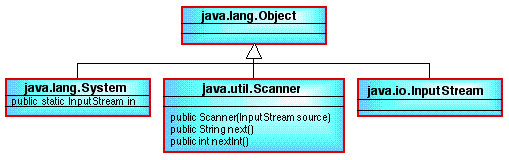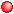# NOTE: This set of www pages is not the set of www pages for the curent version of COMP101. The pages are from a previous version that, at the request of students, I have kept on line.

## CONTENTS

 1. Overview 2. Input 2.1. Example program (Hello World 2) ` ` 3. Numeric Input 4. Formatted output

## 1. OVERVIEW

So far we have only looked at programs which involve output only. There are many applications where we are required to pass some data into the program in the form of input. Here we will examine the mechanism provided by Java to achieve input. The discussion will also serve as a useful revision of the OO features of Java (and OO languages in general).

## 2. INPUTFigure 1: Class diagram showing API classes associated with input

To facilitate input Java provides a class called Scanner. A class diagram indicating the relationship between this class and some other significant classes (at least in the context of input) is given in Figure 1. Note that the classes System and Object are contained in the package java.lang, InputStream in the package java.io, and the class Scanner in the package java.util.

The Scanner class contains (amongst other things) the methods next which is used to input a token. A token, by default, is any sequence of characters delimitted by "while space". Note that a carriage return is required to signal to the Java interpreter that the input is ready for collection.

To use this method we must address two issues:

1. The methods next is an instance methods, thus we require an instance of the Scanner class, i.e. a Scanner object.
2. We must tell Java what the source of the input stream will be, for example the keyboard, secondary storage, Etc.

To create an instance of a class we require an appropriate constructor. Inspection of the Scanner class shows that a constructor of the form:

```Scanner(InputStream source);
```

is provided. The formal parameter to this constructor, source, is an instance of the class (type) InputStream. So we need an instance of the class InputStream. Because input from the keyboard is such a common requirement Java provides an appropriate predefined InputStream object, called in, for keyboard input. This is a class field (keyword static) contained in the class System contained in the package java.lang (which also contains the object out, the instance of the PrintStream class discussed earlier in the context of output) Remember that the package java.lang is always compiled into every Java program. Thus we can create a Scanner object as follows:

` `
```private Scanner input = new Scanner(System.in);
```

Note that:We have called the instance input (we could have called it anything we liked), we say that the object input is an instance of the calss (or is of the type) Scanner.Remember that the keyword new tells the Java compiler to set aside appropriate storage (on the heap) for all the instance fields associated with the class Scanner.Remember also that when we refer to a class field from outside its class we must link it to its class using a membership operator, hence System.in.

Now that we have a Scanner object we can invoke the next method:

```input.next();
```

This is not quite the end of the story, because although next has no formal parameters it returns a string (read from the keyboard) which must be assigned to an appropriately defined variable (data item). We say that the next method has a return type of String. Thus we need a variable of type String in which to store the input. A string variable called name1 would be declared as follows:

```String name1;
```

We can now "capture" keyboard input thus:

```name1 = input.next();
```

Note: the type String is a predefined compound type. The nature of the type String will be discussed further at a later date.

In Figure 2 the input class diagram given in Figure 1 is combined with the output class diagram presented previously.Figure 2: Class diagram showing API classes associated with input and output

### 2.1 Example Program (Hello World 2)

Using the above we can extend the Hello World program described earlier so that it takes some input as indicated by the Java code presented in Table 1.

Note that, in thecode, we have expressly imported the java.util package (unlike java.lang this is not included automatically) using an import statement. The '*' symbol is a "wild card" symbol indicating all the classes in the named package. The java compiler will then "compile in" those classes that are referred to in our class definition. We could have specified particular classes. For example:

```import java.util.Scanner
```

However the effect is the same; there is no performance degradation associated with mentioning the whole package for import hence most programmers use the * wild card character when writing import statements. hence:

```import java.util.*
```
 ```// HALO WORLD PROGRAM 2 // Frans Coenen // Monday 15 January 1999 // Revised Thursday 28 April 2005 for Java 5 // The University of Liverpool, UK // Import packages containing predefined classes import java.util.*; public class HelloWorld2 { // Create instance of Scanner class private static Scanner input = new Scanner(System.in); /** Main method */ public static void main(String[] args) { // Get input System.out.print("What is your name? "); String name1 = input.next(); String name2 = input.next(); // Output System.out.print("\nHello " + name1 + " " + name2); System.out.println(" - Congratulations on writing your first" + " Java program which features some input!\n\n"); } } ```

Table 1: "Hello Word" version 2

## 3. NUMERIC INPUT

In the above example we have shown how we can input a string token into a Java program using the next method. Using this method input is always captured in the form of a string; so what if we wish to input (say) an integer or a float? To do this Java provides a number of specialised methods, one for each primitive type. For example nextInt() returns an int, nextFloat() returns a floating point number (float or a dounle), etc.

The example application program presented in Table 2 illustrates the process of inputting an integer. If (after compilation) we run this program we will be requested for some input, which will then be echoed to the screen as follows:

```\$ java5 IntegerInputApp
Input two integers 23 -47
The values are 23 and -47
```

Try inputting a very large number such as 1000000000000. This will generate an exception because this number is larger than the maximum defined for the integer type:

` `

 ```// INTEGER INPUT // Frans Coenen // Thursday 31 July 2003 // Revised Thursday 28 April 2005 for Java 5 // The University of Liverpool, UK // Import packages containing predefined classes import java.util.*; public class IntegerInputApp { // Create instance of Scanner class private static Scanner input = new Scanner(System.in); /* Main method */ public static void main(String[] args) { // Invite input System.out.print("Input two integers "); // Read in integer int inputInt1 = input.nextInt(); int inputInt2 = input.nextInt(); // Output the result System.out.print("The values are "); System.out.println(inputInt1 + " and " + inputInt2); } } ```

Table 2: Integer input example applications program

```\$ java IntegerInputApp
Input two integers 23 -12345678901234567890
Exception in thread "main" java.util.InputMismatchException: For input string: "-12345678901234567890"
at java.util.Scanner.nextInt(Scanner.java:2046)
at java.util.Scanner.nextInt(Scanner.java:2000)
at IntegerInputApp.main(IntegerInputApp.java:25)

```

Note the InputMismatchException (do not worry at this stage if you do not understand all of the resulting output.)

## 4. FORMATTED OUTPUT

Instead of the output given in Tables 1 and 2:

```System.out.print("\nHello " + name1 + " " + name2);
System.out.println(inputInt1 + " and " + inputInt2);
```

We could have used the printf method from the PrintStream class, and written:

```System.out.printf("\nHello %s %s",name1,name2);
System.out.printf("%d and %d\n",inputInt1,inputInt2);
```

(The efeect would be the same.) The printf ("print formatted") method can have any number of arguments the first of which must be a format sting. The format string will include format specifiers, one for each of the remaining arguments, which specify how each argument should be output. Format specifiers typically comprise the following:

1. A % character (operator) that tells Java that what follows is a format specifier.
2. An (optional) flag indicating whether (say) the argument should be left justified (arguments are right justified by default) or zero padded or include a sign etc. Some flags are only appropriate with respect to numeric output.
3. An (optional) width indicating the minimum number of characters to be written to the output.
4. The type of the output, common examples include: s = string, c = character, d = decimal integer (as opposed to say octel, hexidecimal etc.) and f = floating point.

If we replace the output statements included in Table 2 with:

```System.out.print("The values are: ");
System.out.printf("%010d and %010d\n",inputInt1,inputInt2);
System.out.printf("%-10d\n",inputInt1);
System.out.printf("%-10d\n",inputInt2);
System.out.printf("%10d\n",inputInt1);
System.out.printf("%10d\n",inputInt2);
```

and run the application again we will get:

```\$ java IntegerInputApp
Input an integer 23 56789
The values are: 0000000023 and 0000056789
23
56789
23
56789
```

Further information regarding format stings can be obtained from the the Sun Java WWW site.

Created and maintained by Frans Coenen. Last updated 10 February 2015